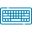# Maclaurin Series Calculator## Table of Content

Feedback

Find the expansion of a given function at a given point with this Maclaurin series calculator. This tool expands the Taylor series so that it becomes zero for the given function, with the steps shown.

## What is the Maclaurin Series?

In Mathematics,

The Maclaurin series is a type of Taylor series where the center point is always set to be a = 0. We can start from any point in the Taylor series, but in the Maclaurin calculator series, it's always zero.

The Maclaurin series is like an expanded series of a function that shows a function more clearly, aiming for an ideal representation that closely resembles the original function. You might find it useful in engineering, statistics, physics, or actuarial work.

## Maclaurin Series Formula:

Maclaurin series calculator computes a series expansion for any function using the following formula:

$$f(x) = f(0) + f'(0)x + f''(0)x²/2! + f'''(0)x³/3! + ...$$

In this formula,

The function f’(x), f’’(x), and f’’’(x) represents the first derivative, second derivative, and third derivative of the function evaluated at the value of x = 0, respectively.

## How to calculate the Maclaurin series?

To manually find MacLaurin series for a function, perform the following steps:

• Determine the function's derivatives: Determine the first derivative, second derivative, third derivative, etc. of the function with the variable x.
• Evaluate the value of derivatives at x = 0.
• Write the Maclaurin series: The Maclaurin series can be constructed by taking the derivative values at x = 0, and the factorial function of the sequence of each term.

The basic formula for the Maclaurin series is:

$$f(x) = f(0) + f'(0)x + f''(0)x²/2! + f'''(0)x³/3! + …$$

Let’s now look at the example to show you how to manually calculate the Maclaurin series:

### Example:

Find the Maclaurin series for f(x) = e^x up to 4th order.

Derivatives:

f(x) = e^x, f'(x) = e^x, f''(x) = e^x, f'''(x) = e^x, f⁴(x) = e^x.

Determine the derivatives at x = 0:

f(0) = e^0 = 1, f'(0) = e^0 = 1, f''(0) = e^0 = 1, f'''(0) = e^0 = 1, f⁴(0) = e^0 = 1.

Write down the Maclaurin series up to the 4th order:

⇒ f(x) ≈ 1 + x + x²/2! + x³/3! +...

Here’s the final output for the Maclaurin series for cos x.

## How Maclaurin Series Calculator Works?

Here is how the Maclaurin polynomial calculator calculates the expansion series for any function:

Input:

• First, enter the complete equation/function
• Enter the order number which you want to calculate
• Press the Calculate Button

Output:

• A window will appear with the final result of the expansion series for the given function

## FAQs:

### Why is it called the Taylor and Maclaurin series?

In the case where the center of the Taylor series lies at zero, the resulting series is also referred to as the MacLaurin series, named after the 18th-century Scottish mathematician, Colin MacLaurin. MacLaurin made great use of this particular case of the Taylor series.

### What are the limitations of the Maclaurin series?

There are some limitations of the Maclaurin series such as:

• It becomes complicated to derive successive terms.
• The truncation error increases rapidly when the expansion point is reached.
• It is rarely as efficient as curve fitting or direct approximation.

### What are the applications of the Maclaurin series?

Maclaurin expansions have many applications which include:

•  Evaluating definite integrals.
•  Finding the limit of a function.
•  Approximating the value of an expression.

## References:

From the source Wikipedia: Taylor series, Definition, History, and Analytic functions.### Hamza Haroon

I am a blogger, researcher, technical content writer and science geek who writes on Science & Technology. My hobbies includes collecting old coins, reading books and watching detective series. I love football and seeing Real Madrid play in traditional